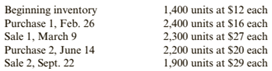Chapter 6, Problem 63E### Cornerstones of Financial Accounti...

4th Edition
Jay Rich + 1 other
ISBN: 9781337690881

#### Solutions

Chapter
Section### Cornerstones of Financial Accounti...

4th Edition
Jay Rich + 1 other
ISBN: 9781337690881
Textbook Problem
75 views

# ( Appendix 6B) Inventory Costing Methods: Periodic Inventory SystemThe inventory accounting records for Lee Enterprises contained the following data:Required:1. Calculate the cost of ending inventory and the cost of goods sold using the FIFO, LIFO, and average cost methods. ( Note: Use four decimal places for per-unit calculations and round all other numbers to the nearest dollar.)2. CONCEPTUAL CONNECTION Compare the ending inventory and cost of goods sold computed under all three methods. What can you conclude about the effects of the inventory costing methods on the balance sheet and the income statement?

To determine

(a)

Inventory costing methods:

FIFO, LIFO and average cost method, are those method which used for calculation of closing inventory and cost of goods sold.

The cost of ending inventory and the cost of goods sold using the three methods.

Explanation

Periodic inventory system:

Under periodic inventory system, quantity and value of sales are recorded and sales are recorded only on value basis thus quantity of cost of goods sold and closing inventory was not available. At the year end, we calculate closing inventory with physical verification of stock and subtract with available quantity to determine actual quantity sold.

Total available units are:

Opening inventory 1400 units @ $12 each Purchases=2400 units @$16 eachPurchases=2200 units @$20 each Total available quantity = 1400+2400+2200=6000 units Sales(March, 9)=2300 units @$27 eachSales(Sept., 22)=1900 units @$29 each Calculation of Closing Inventory as per FIFO Method: Under this method, which material purchased first, issued first for production. However closing inventory includes last purchased materials in stock. Due to latest purchase in closing inventory, higher value of latest purchase effects cost of goods sold as lower and profit margin will be high.  Closing inventory Cost of Goods sold 1400 @$12 = $16800 900 @$16 = $14400 1500 @$16 = $24000 1800 @$20 = $36000 400 @$20 = 8000 Total 1800 @$20 =$36000 4200 units = \$63200

Calculation of closing inventory as per LIFO Method:

Under this method, which material purchased last, issued first for production. However closing inventory includes earliest purchased material in stock

To determine

(b)

Inventory costing methods:

FIFO, LIFO and average cost method, are those method which used for calculation of closing inventory and cost of goods sold.

The comparison between cost of ending inventory and the cost of goods sold using the three methods.

### Still sussing out bartleby?

Check out a sample textbook solution.

See a sample solution

#### The Solution to Your Study Problems

Bartleby provides explanations to thousands of textbook problems written by our experts, many with advanced degrees!

Get Started

#### What is the primary value of a firewall?

Principles of Information Security (MindTap Course List)

#### Why is tire rotation recommended by most manufacturers?

Automotive Technology: A Systems Approach (MindTap Course List)

#### What do we mean by nominal and effective interest rates?

Engineering Fundamentals: An Introduction to Engineering (MindTap Course List)